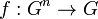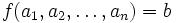# Multiary group

This is a variation of group|Find other variations of group | Read a survey article on varying group
QUICK PHRASES: group-like structure with n-ary instead of binary operation for multiplication

## Definition

A multiary group or polyadic group is a$n$-ary group for some$n \ge 2$. Note that the$n = 2$ case corresponds to the usual notion of group.

A$n$-ary group is defined as a set$G$ with a$n$-ary operation, i.e., a map$f: G^n \to G$ such that:

1. It is a multiary semigroup: All different ways of associating expressions involving the$n$-ary operation$f$ yield equivalent results. Note that this boils down to checking that all the$n$ distinct possible ways of associating an expression of length$2n - 1$ give the same answer.
2. It is a multiary quasigroup: The equation$f(a_1,a_2,\dots,a_n) = b$ has a unique solution with any$a_i$ as the unknown and all other quantities known.

Note that we do not assume separate axioms on identity and inverses. Rather, we bundle both into the unique solution condition (part (2) of the definition). To see why$n = 2$ gives a group, refer associative quasigroup implies group.

## Related notions

• Multiary semigroup: This only requires condition (1), the associativity condition.
• Multiary quasigroup: This only requires condition (2), the unique solutions condition.
• Hypergroup: A different type of variation, where the multiplication operation is still binary, but can now give multiple outputs.

## Facts

### A group gives a$n$-ary group for all$n$

Further information: Group is n-ary group for all n

The structure of a group on a set also equips with the structure of a$n$-ary group for any$n$. For this, we define the$n$-ary product to simply be the product of the$n$ elements (in that particular sequence) in the group. Briefly:

• The product is well-defined independent of parenthesization because of the associativity of the group multiplication.
• The associativity condition for the$n$-ary group follow from those for a group.
• The unique solution condition follows from the fact that these conditions hold in groups. Further information: Group implies quasigroup, manipulating equations in groups

### Reducibility and irreducibility

Not every$n$-ary group arises from a group in this way. A$n$-ary group that arises from a group is termed reducible, and a$n$-ary group that does not arise from a group in this way is termed irreducible. It turns out that a$n$-ary group is reducible if and only if its set of neutral elements is non-empty.